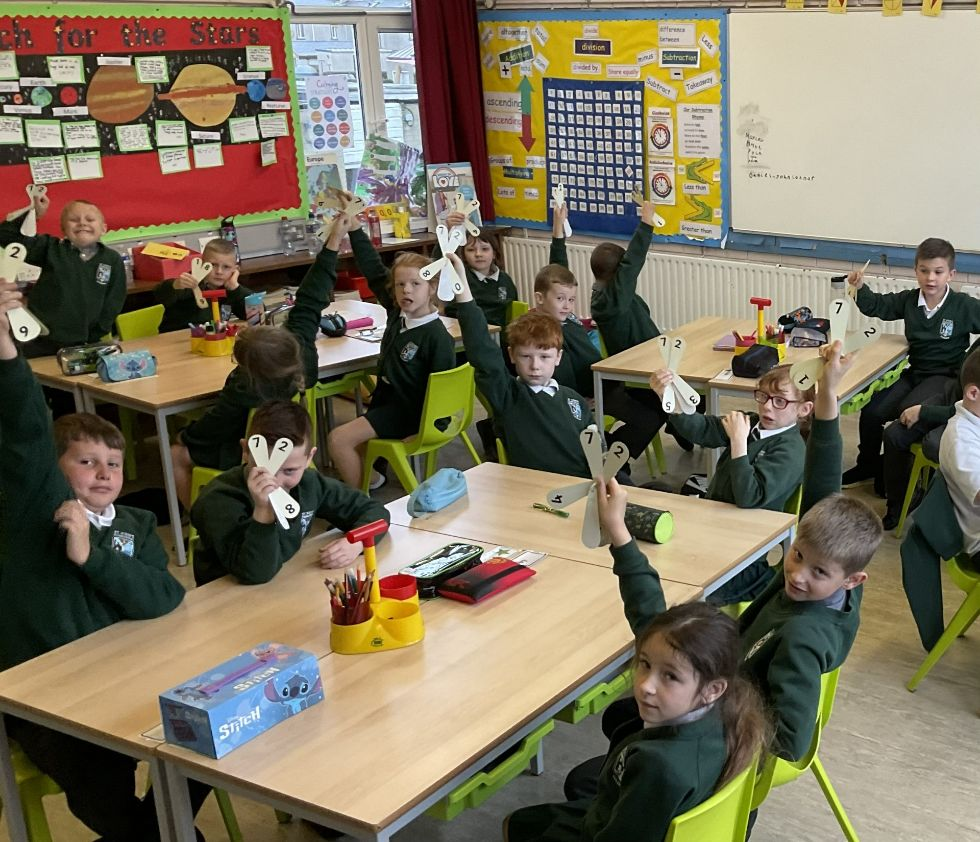## Maths & NumeracyUsing magnetic polydron to investigate nets of 3D shapes

Using magnetic polydron to investigate nets of 3D shapes

Using magnetic polydron to investigate nets of 3D shapes

Building a Marble Run

Building a Marble Run

This area of learning can be sub-divided into Number, Measure, Shape and Space and Handling Data. In each of these sub-divisions, children use practical resources to develop and consolidate their understanding of mathematical and numerical concepts. Teachers use mathematical vocabulary when introducing and modelling mathematical learning. Children are encouraged to use mathematical vocabulary when explaining their calculations and understanding. We present mathematical learning in relevant and meaningful contexts for our children and place emphasis on problem solving to challenge and extend our children’s understanding.

Children in Years 4 to 7 are enrolled in Mathletics which is an online mathematical programme that provides students with online tutorials which adapt to meet the needs of each individual child. Children demonstrate their learning by answering questions which in turn allows them to earn points to reach either Bronze, Silver or Gold Award Certificates. At the end of each term, we present certificates to children who have reached these points at a special assembly.